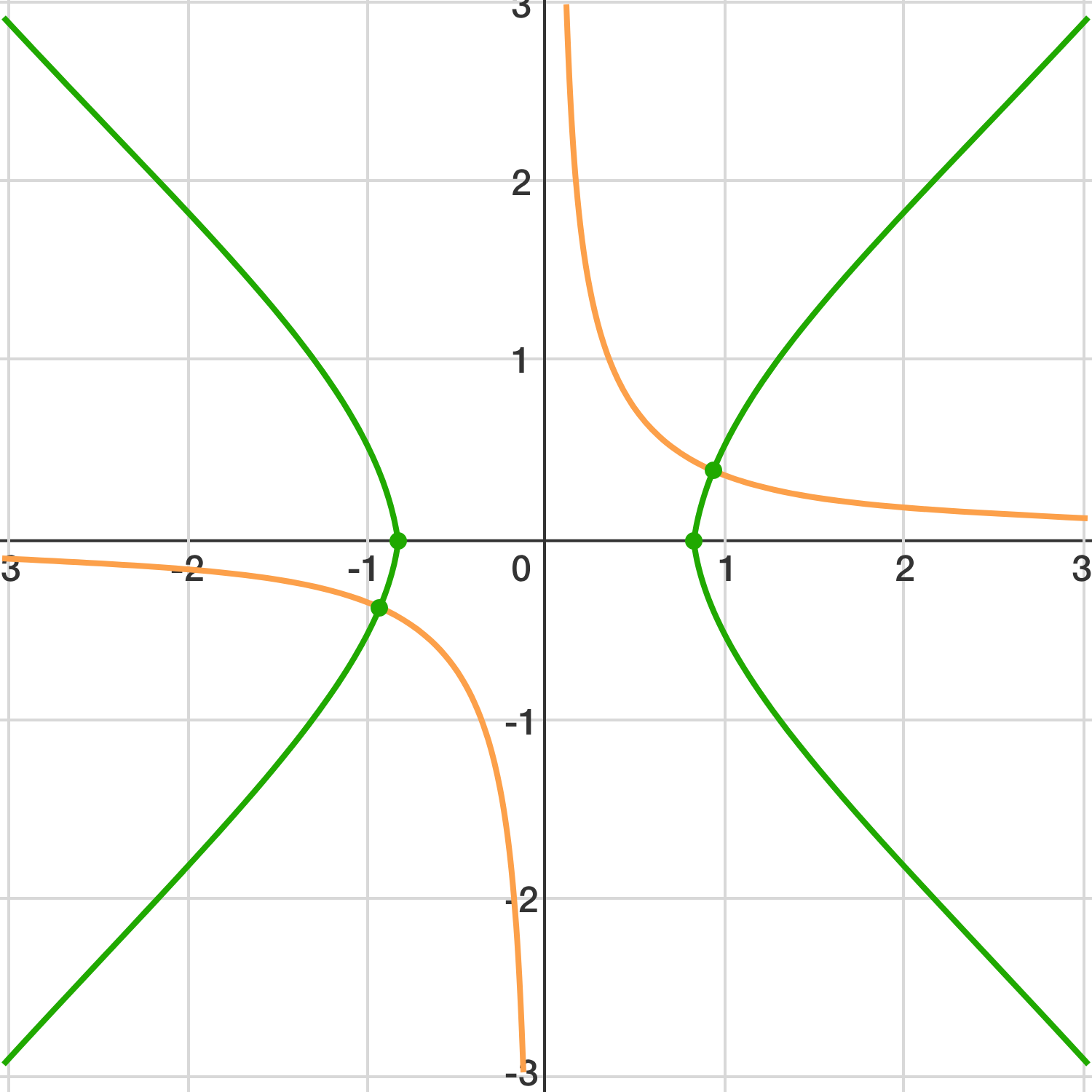# Rotating the Hyperbola

Geometry Level 4A hyperbola $H$ with equation $xy=n$ (where $n\le 1000$) is rotated $45^{\circ}$ to obtain the hyperbola $H'$. Let the positive difference between the number of lattice points on $H$ and $H'$ be $D$. Given that both $H$ and $H'$ have at least one lattice point, find the maximum possible value of $D$.

Details and Asumptions: A lattice point is a point that has integer $x$- and $y$-coordinates.

You may want to look at the list of Highly Composite Numbers.

×